TitleCollege Algebra
Tutorial 47: Exponential Growth and DecayLearning Objectives

 After completing this tutorial, you should be able to: Solve exponential growth problems. Solve exponential decay problems.Introduction

 In this tutorial I will step you through how to solve problems that deal in exponential growth and decay.  These problems will require you to know how to evaluate exponential expressions and solve exponential equations.  If you need a review on these topics, feel free to go to Tutorial 42: Exponential Functions and Tutorial 45: Exponential Equations.  Ready, set, GO!!!!!Tutorial

 Exponential Growth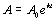,   (k > 0)

 A represents the amount at a given time t. Ao represents the initial amount of the growing entity.  Note that this is the amount when t = 0. k is a constant that represents the growth rate.  It is POSITIVE when talking in terms of exponential GROWTH. t is the amount of time that has past.  If the information for time is given in dates, you need to convert it to how much time has past since the initial time.  For example, if the model is set up at an initial year of 2000 and you need to find out what the value is in the year 2010, t would be 2010 - 2000 = 10 years. You can use this formula to find any of its variables, depending on the information given and what is being asked in a problem.  For example, you may be given the values for Ao and t and you need to find the amount A after the given time.  Or, you may be given the final amount A and the initial amount Ao and you need to find the time t. Some examples of exponential growth are population growth and financial growth.  The information found, can help predict what a population for a city or colony would be in the future or what the value of your house is in ten years.  Or you can use it to find out how long it would take to get to a certain population or value on your house. The diagram below shows exponential growth: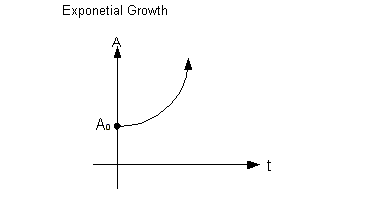Example 1: The exponential growth modeldescribes the population of a city in the United States, in thousands, t years after 1994.  Use this model to solve the following: A) What was the population of the city in 1994? B) By what % is the population of the city increasing each year? C) What will the population of the city be in 2005? D) When will the city’s population be 60 thousand?

 A) What was the population of the city in 1994?

 Since we are looking for the population, what variable are we seeking?  If you said A you are right on!!!! The way the problem is worded, 1994 is what we call our initial year.  This is when t = 0.  Plugging in 0 for t and solving for A we get:*Replace t with 0 *e^0 simplifies to be 1

 When writing up the final answer, keep in mind that the problem said that the population was in thousands.  The population in 1994 would be 30,000. Another way that we could have approached this problem was noting that the year was 1994, which is our initial year, so basically it was asking us for the initial population, which is Ao in the formula.  This happens to be the number in front of e which is 30 in this problem.   The reason I showed you using the formula was to get you use to it.  Just note that when it is the initial year, t is 0, so you will have e raised to the 0 power which means it will simplify to be 1 and you are left with whatever Ao is.

 B) By what % is the population of the city increasing each year?

 As mentioned above, in the general growth formula, k is a constant that represents the growth rate. k is the coefficient of t in e’s exponent. So what would be our answer in terms of percent? Well, k = .0198026, so converting that to percent we get 1.98026% for our answer.

 C) What will the population of the city be in 2005?

 Since we are looking for the population, what variable are we finding?  If you said A give yourself a high five. What are we going to plug in for t in this problem? Our initial year is 1994, and since t represents years after 1994, we can get t from 2005 - 1994, which would be 11. Plugging in 11 for t and solving for A we get: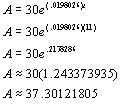*Replace t with 11 *e^ .2178286 is approx. 1.243373935

 Again, when writing up the final answer, keep in mind that the problem said that the population was in thousands.  The population in 2005 would be approximately 37, 301.

 D) When will the city’s population be 60 thousand?

 Looks like we have a little twist here.  Now we are given the population and we need to first find t to find out how many years after 1994 we are talking about and then convert that knowledge into the actual year. We will still be using the same formula we did to answer the questions above, we will just be using it to find a different variable. Plugging in 60 for A and solving for t we get: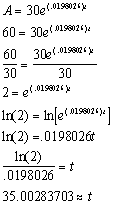*Replace A with 60 *Take the ln of both sides *Use Inv Prop I of Logs to simplify

 This means a little over 35 years after 1994, the population will be 60 thousand. So our answer is during the year 2029.Example 2: A house is purchased for \$150,000 in 2002. The value of the house is given by the exponential growth model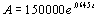.  Find when the house would be worth \$200,000.

 Since we are looking for when, what variable do we need to find?   If you said t give yourself a high five.      What are we going to plug in for A in this problem?  If you said 200000, you are correct! Plugging 200000 for A in the model we get: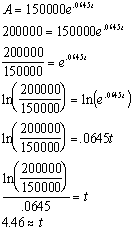*Replace A with 20000 *Take the ln of both sides *Use Inv Prop I of Logs to simplify

 Rounding 4.46 to the nearest whole number we get t = 4.  Since t is measured in years since 2002, the model indicates that the population will reach 200000 in year 2002 + 4 = 2006.

 Exponential Decay,   (k < 0)

 A represents the amount at a given time t. Ao represents the initial amount of the growing entity.  Note that this is the amount when t = 0. k is a constant that represents the growth rate.  It is NEGATIVE when talking in terms of exponential DECAY. t is the amount of time that has past.  If the information for time is given in dates, you need to convert it to how much time has past since the initial time.  For example, if the model is set up at an initial year of 2000 and you need to find out what the value is in the year 2010, t would be 2010 - 2000 = 10 years. You can use this formula to find any of its variables, depending on the information given and what is being asked in a problem.  For example, you may be given the values for Ao and t and you need to find the amount A after the given time.  Or, you may be given the final amount A and the initial amount Ao and you need to find the time t. Examples of exponential decay are radioactive decay and population decrease.  The information found can help predict what the half-life of a radioactive material is or what the population will be for a city or colony in the future.  The half-life of a given substance is the time required for half of that substance to decay or disintegrate. The diagram below shows exponential decay: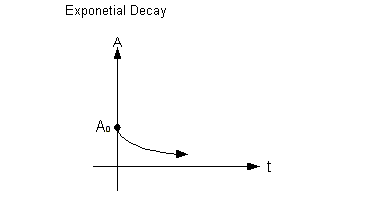Example 3: An artifact originally had 12 grams of carbon-14 present.  The decay model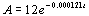describes the amount of carbon-14 present after t years.  How many grams of carbon-14 will be present in this artifact after 10,000 years?

 If we are looking for the number of grams of carbon-14 present, what variable do we need to find?  If you said A give yourself a high five. What are we going to plug in for t in this problem? Since t represents the number of years, it looks like we will be plugging in 10,000 for t. Plugging in 10000 for t and solving for A we get: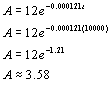*replace t with 10000

 There will be approximately 3.58 grams of carbon-14 present after 10,000 years.Example 4: A certain radioactive isotope element decays exponentially according to the model, where A is the number of grams of the isotope at the end of t days and Ao is the number of grams present initially.  What is the half-life of this isotope?

 If we are looking for the half-life of this isotope, what variable are we seeking?  If you said t you are correct!!!!  It looks like we don’t have any values to plug into A or Ao.  However, the problem did say that we were interested in the HALF-life, which would mean ½ of the initial amount (Ao) would be present at the end (A) of that time.  This means A can be replaced with .5Ao. Replacing A with .5 Ao and solving for t we get: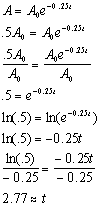*Replace A with .5Ao *Divide both sides by Ao *Take the ln of both sides *Use Inv Prop I of Logs to simplify

 The half-life of this isotope is approximately 2.77 days.Example 5: Prehistoric cave paintings were discovered in a cave in Egypt.  The paint contained 20% of the original carbon-14.  Using the exponential decay model for carbon-14,, estimate the age of the paintings.

 Since we are looking for the age of the paintings, what variable are we looking for?  If you said t you are correct!!!! It looks like we don’t have any values to plug into A or Ao.  However, the problem did say that the paintings that were found contained 20% of the original carbon-14.  This means A can be replaced with .2Ao (20% of the original). Replacing A with .2 Ao and solving for t we get: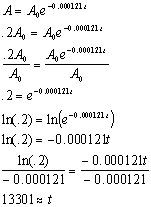*Replace A with .2Ao *Divide both sides by Ao *Take the ln of both sides *Use Inv Prop I of Logs to simplify

 The age of the paintings are approximately 13301 years.Practice Problems

 These are practice problems to help bring you to the next level.  It will allow you to check and see if you have an understanding of these types of problems. Math works just like anything else, if you want to get good at it, then you need to practice it.  Even the best athletes and musicians had help along the way and lots of practice, practice, practice, to get good at their sport or instrument.  In fact there is no such thing as too much practice. To get the most out of these, you should work the problem out on your own and then check your answer by clicking on the link for the answer/discussion for that  problem.  At the link you will find the answer as well as any steps that went into finding that answer.Practice Problems 1a - 1b:   Solve the given exponential growth or decay problem.

 1a.  The value of the property in a particular block follows a pattern of exponential growth.  In the year 2001, your company purchased a piece of property in this block.  The value of the property in thousands of dollars, t years after 2001 is given by the exponential growth model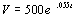. Use this model to solve the following: A) What did your company pay for the property? B) By what percentage is the price of the property in this block increasing per year? C) What will the property be worth in the year 2010? D) When will the property be worth 750 thousand dollars? (answer/discussion to 1a)

 1b. An artifact originally had 10 grams of carbon-14 present.  The decay modeldescribes the amount of carbon-14 present after t years.  Use this model to solve the following: A) How many grams of carbon-14 will be present in this artifact after 25,000 years? B) What is the half-life of carbon-14? (answer/discussion to 1b)Need Extra Help on these Topics?

The following are webpages that can assist you in the topics that were covered on this page.

 http://www.purplemath.com/modules/expoprob2.htm This webpage will help you with exponential growth problems. http://www.purplemath.com/modules/expoprob3.htm This webpage will help you with exponential decay problems.

Go to Get Help Outside the Classroom found in Tutorial 1: How to Succeed in a Math Class for some more suggestions.

Last revised on March 25, 2011 by Kim Seward.
All contents copyright (C) 2002 - 2011, WTAMU and Kim Seward. All rights reserved.